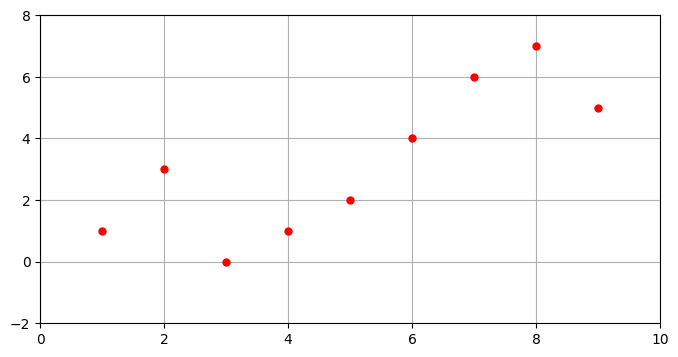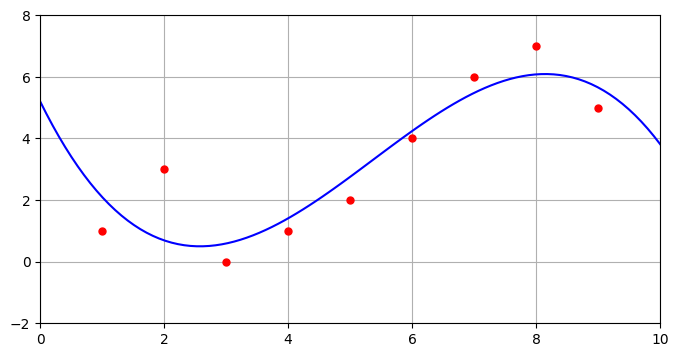# Polynomial regression¶

In :
# define (x,y) coordinates of the points
x = [ 1, 2, 3, 4, 5, 6, 7, 8, 9 ]
y = [ 1, 3, 0, 1, 2, 4, 6, 7, 5 ]

using PyPlot
figure(figsize=(8,4))
plot(x,y,"r.", markersize=10)
axis([0,10,-2,8])
grid("on")In :
# order of polynomial to use
k = 3

# fit using a function of the form f(x) = u1 x^k + u2 x^(k-1) + ... + uk x + u{k+1}
n = length(x)
A = zeros(n,k+1)
for i = 1:n
for j = 1:k+1
A[i,j] = x[i]^(k+1-j)
end
end

In :
# NOTE: must have either Gurobi or Mosek installed!

using JuMP, Gurobi

#m = Model(solver=MosekSolver(LOG=0))
m = Model(solver=GurobiSolver(OutputFlag=0))
#m = Model(solver=GurobiSolver(OutputFlag=1,NumericFocus=2))    # extra option to do extra numerical conditioning
#m = Model(solver=GurobiSolver(OutputFlag=1,BarHomogeneous=1))  # extra option to use alternative algorithms

@variable(m, u[1:k+1])
@objective(m, Min, sum( (y - A*u).^2 ) )

status = solve(m)
uopt = getvalue(u)
println(status)

Academic license - for non-commercial use only
Optimal

In :
uopt

Out:
4-element Array{Float64,1}:
-0.0648148
1.04257
-4.08309
5.20635  
In :
inv(A'*A)*(A'*y)

Out:
4-element Array{Float64,1}:
-0.0648148
1.04257
-4.08309
5.20635  
In :
A\y

Out:
4-element Array{Float64,1}:
-0.0648148
1.04257
-4.08309
5.20635  
In :
using PyPlot
npts = 100
xfine = linspace(0,10,npts)
ffine = ones(npts)
for j = 1:k
ffine = [ffine.*xfine ones(npts)]
end
yfine = ffine * uopt
figure(figsize=(8,4))
plot( x, y, "r.", markersize=10)
plot( xfine, yfine, "b-")
axis([0,10,-2,8])
grid()In :
# NOTE: problem can be solved using ECOS or SCS if written as an "SOCP" --- more on this later!
# Here is a working example:

using JuMP, ECOS, SCS

#m = Model(solver=ECOSSolver(verbose=false))
m = Model(solver=SCSSolver(verbose=false))

@variable(m, u[1:k+1])
@variable(m, t)
@constraint(m, norm(y - A*u) <= t)
@objective(m, Min, t)

status = solve(m)
uopt = getvalue(u)
println(status)
println(uopt)

Optimal
[-0.0648149, 1.04257, -4.0831, 5.20635]

In [ ]: